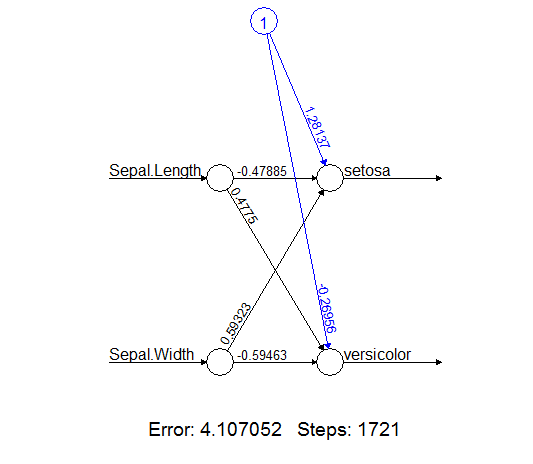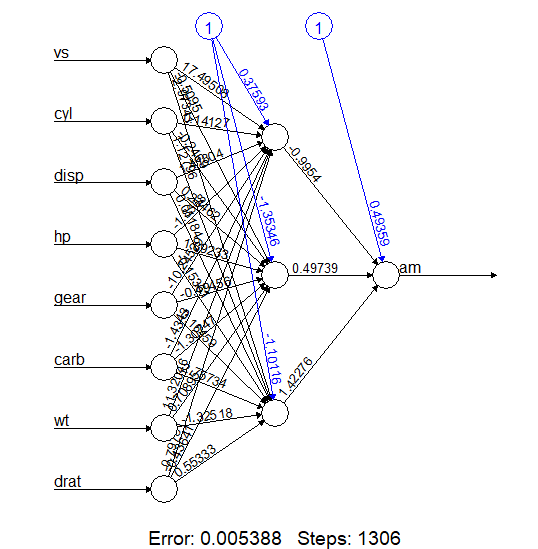# Single Layered Neural Networks in R Programming

• Last Updated : 22 Jul, 2020

Neural networks also known as neural nets is a type of algorithm in machine learning and artificial intelligence that works the same as the human brain operates. The artificial neurons in the neural network depict the same behavior of neurons in the human brain. Neural networks are used in risk analysis of business, forecasting the sales, and many more. Neural networks are adaptable to changing inputs so that there is no need for designing the algorithm again based on the inputs. In this article, we’ll discuss single-layered neural network with its syntax and implementation of the `neuralnet()` function in R programming. Following function requires `neuralnet` package.

#### Types of Neural Networks

Neural Networks can be classified into multiple types based on their depth activation filters, Structure, Neurons used, Neuron density, data flow, and so on. The types of Neural Networks are as follows:

1. Perceptron
2. Feed Forward Neural Networks
3. Convolutional Neural Networks
4. Radial Basis Function Neural Networks
5. Recurrent Neural Networks
6. Sequence to Sequence Model
7. Modular Neural Network

Depending upon the number of layers, there are two types of neural networks:

1. Single Layered Neural Network: A single layer neural network contains input and output layer. The input layer receives the input signals and the output layer generates the output signals accordingly.
2. Multilayer Neural Network: Multilayer neural network contains input, output and one or more than one hidden layer. The hidden layers perform intermediate computations before directing the input to the output layer.

#### Single Layered Neural Network

A single-layered neural network often called perceptrons is a type of feed-forward neural network made up of input and output layers. Inputs provided are multi-dimensional. Perceptrons are acyclic in nature. The sum of the product of weights and the inputs is calculated in each node. The input layer transmits the signals to the output layer. The output layer performs computations. Perceptron can learn only a linear function and requires less training output. The output can be represented in one or two values(0 or 1).

#### Implementation in R

R language provides `neuralnet()` function which is available in `neuralnet` package to perform single layered neural network.

Syntax:
neuralnet(formula, data, hidden)

Parameters:
formula: represents formula on which model has to be fitted
data: represents dataframe
hidden: represents number of neurons in hidden layers

To know about more optional parameters of the function, use below command in console: help(“neuralnet”)

Example 1:
In this example, let us create the single-layered neural network or perceptron of iris plant species of setosa and versicolor based on sepal length and sepal width.
Step 1: Install the required package

 `# Install the required package ` `install.packages``(``"neuralnet"``) `

Step 2: Load the package

 `# Load the package ` `library``(neuralnet) `

Step 3: Load the dataset

 `# Load dataset ` `df <- iris[1:100, ] `

Step 4: Fitting neural network

 `nn = ``neuralnet``(Species ~ Sepal.Length  ` `              ``+ Sepal.Width, data = df, ` `              ``hidden = 0, linear.output = ``TRUE``) `

Step 5: Plot neural network

 `# Output to be present as PNG file ` `png``(file = ``"neuralNetworkGFG.png"``) ` ` `  `# Plot ` `plot``(nn) ` ` `  `# Saving the file ` `dev.off``() `

Output:Example 2:
In this example, let us create more reliable neural network using multi-layer neural network and make predictions based on the dataset.
Step 1: Install the required package

 `# Install the required package ` `install.packages``(``"neuralnet"``) `

Step 2: Load the package

 `# Load the package ` `library``(neuralnet) `

Step 3: Load the dataset

 `# Load dataset ` `df <- mtcars `

Step 4: Fitting neural network

 `nn <- ``neuralnet``(am ~ vs + cyl + disp + hp + gear  ` `                ``+ carb + wt + drat, data = df,  ` `               ``hidden = 3, linear.output = ``TRUE``) `

Step 5: Plot neural network

 `# Output to be present as PNG file ` `png``(file = ``"neuralNetwork2GFG.png"``) ` ` `  `# Plot ` `plot``(nn) ` ` `  `# Saving the file ` `dev.off``() `

Step 6: Create test dataset

 `# Create test dataset ` `vs = ``c``(0, 1, 1) ` `cyl =``c``(6, 8, 8) ` `disp = ``c``(170, 250, 350) ` `hp = ``c``(120, 240, 300) ` `gear = ``c``(4, 5, 4) ` `carb = ``c``(4, 3, 3) ` `wt = ``c``(2.780, 3.210, 3.425) ` `drat = ``c``(3.05, 4.02, 3.95) ` ` `  `test <- ``data.frame``(vs, cyl, disp, hp,  ` `                  ``gear, carb, wt, drat) `

Step 7: Make prediction of test dataset

 `Predict <- ``compute``(nn, test) ` ` `  `cat``(``"Predicted values:\n"``) ` `print``(Predict\$net.result) `

Step 8: Convert prediction into binary values

 `probability <- Predict\$net.result ` `pred <- ``ifelse``(probability > 0.5, 1, 0) ` `cat``(``"Result in binary values:\n"``) ` `print``(pred) `

Output:```Predicted values:
[,1]
[1,] 0.3681382
[2,] 0.9909768
[3,] 0.9909768

Result in binary values:
[,1]
[1,]    0
[2,]    1
[3,]    1
```

Explanation:
In above output, “am” value for each row of test dataset is predicted using multi-layer neural network. As in neural network created by the function, predicted values greater than 0.49 makes “am” value of car to be 1.

#### Advantages of Single-layered Neural Network

• Single layer neural networks are easy to set up and train them as there is absence of hidden layers
• It has explicit links to statistical models

#### Disadvantages of Single-layered Neural Network

• It can work better only for linearly separable data.
• Single layer neural network has low accuracy as compared to multi-layer neural network.

My Personal Notes arrow_drop_up
Recommended Articles
Page :